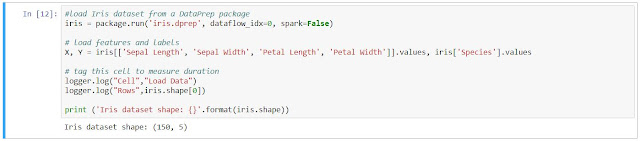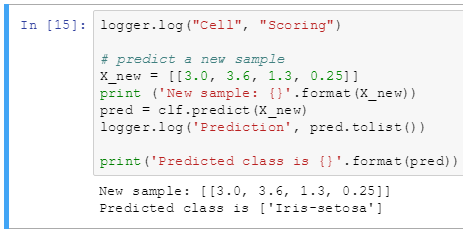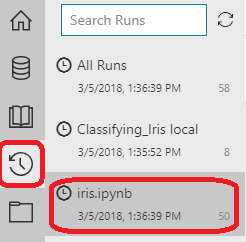## Monday, June 4, 2018

### Azure Machine Learning Workbench: Classifying Iris Data using Python

Today, we're going to finish our walkthrough of the "Classifying_Iris" template provided as part of the AML Workbench.  Previously, we've looked at Getting Started, Utilizing Different Environments, Built-In Data Sources, Built-In Data Preparation (Part 1, Part 2, Part 3) and Python Notebooks.  In this post, we're going to finish looking through the "iris" notebook.  If you haven't read the previous post, it's recommended that you do so as it provides necessary context around Jupyter Notebooks.

Let's jump straight into the first code segment.Segment 1
For those that can't read this, here's the code:

<CODE START>

#load Iris dataset from a DataPrep package
iris = package.run('iris.dprep', dataflow_idx=0, spark=False)

# load features and labels
X, Y = iris[['Sepal Length', 'Sepal Width', 'Petal Length', 'Petal Width']].values, iris['Species'].values

# tag this cell to measure duration
logger.log("Cell","Load Data")
logger.log("Rows",iris.shape)

print ('Iris dataset shape: {}'.format(iris.shape))

<CODE END>

This code segment starts by using the azureml.dataprep.run() function, aliased as package.run(), to retrieve the iris dataset that was prepared using the Built-In Data Preparation tools within AML Workbench.  To learn more about this, please see the previous posts in this series (Part 1Part 2Part 3).

A very interesting feature of this function is that the azureml library is available to ANY Python IDE.  This means that we can build the dataset once using the ease and flexibility of AML Workbench, but still share the data with our colleagues who may want to use other IDEs.

Once the data is imported, we run the following line:

X, Y = iris[['Sepal Length', 'Sepal Width', 'Petal Length', 'Petal Width']].values, iris['Species'].values

This line can be effectively thought of as two commands, wrapped up in a single line.

X = iris[['Sepal Length', 'Sepal Width', 'Petal Length', 'Petal Width']].values
Y = iris['Species'].values

Basically, these commands create two NumPy ndarray objects, X and Y.  A NumPy ndarray object is a multidimensional object containing a series of elements of the SAME TYPE, i.e. numbers, strings, etc.  An ndarray can be as large or as complex as we like, so long as we have enough RAM to hold it.  To learn more about ndarrays, read this.

In this case, X is a two-dimensional ndarray (think "rows and columns") populated with the features "Sepal Length", "Sepal Width", "Petal Length" and "Petal Width".  Y is a one-dimensional ndarray (think "single column") populated with the label "Species".

The next two lines of code are as follows:

logger.log("Cell","Load Data")
logger.log("Rows",iris.shape)

These lines simply output values to the Run History.  We'll take a look at this at the end of this post.

The final line of this code segment is below:

print ('Iris dataset shape: {}'.format(iris.shape))

This line simply outputs the dimensions of the iris ndarray.  We saw this in the picture earlier.  Let's take a look at the next code segment.Segment 2
Here's the code for Segment 2:

<CODE START>

logger.log("Cell", "Training")

# change regularization rate and you will likely get a different accuracy.
reg = 0.01

print("Regularization rate is {}".format(reg))
logger.log('Regularization Rate', reg)

# train a logistic regression model
clf = LogisticRegression(C=1/reg).fit(X, Y)
print (clf)

# Log curves for label value 'Iris-versicolor'
y_scores = clf.predict_proba(X)
precision, recall, thresholds = precision_recall_curve(Y, y_scores[:,1],pos_label='Iris-versicolor')
logger.log("Precision",precision)
logger.log("Recall",recall)
logger.log("Thresholds",thresholds)

accuracy = clf.score(X, Y)
logger.log('Accuracy', accuracy)
print ("Accuracy is {}".format(accuracy))

<CODE END>

The first line is below:

logger.log("Cell", "Training")

Like the previous lines, this one outputs more information to the Run History.  Here are the next few lines:

reg = 0.01

print("Regularization rate is {}".format(reg))
logger.log('Regularization Rate', reg)

These lines define the Regularization Rate.  Regularization is a method designed to reduce overfitting.  The goal of regularization is to cause the model training process to stop once it has reach a "good enough" solution.  This reduces the chance that the model will train to the point of being extremely accurate at predicting the values in the training set, but not as accurate at predicting new values.  To learn more, check out the pages linked above.

These lines also output the Regularization Rate to the notebook and the Run History.  Let's check out the next couple of lines.

clf = LogisticRegression(C=1/reg).fit(X, Y)
print (clf)

The first line fits a Logistic Regression model to predict the values in Y using the values in X as predictors.  The next line outputs the model to the notebook so that we can see the full parameter list for the model.  Let's move on.

y_scores = clf.predict_proba(X)
precision, recall, thresholds = precision_recall_curve(Y, y_scores[:,1],pos_label='Iris-versicolor')
logger.log("Precision",precision)
logger.log("Recall",recall)
logger.log("Thresholds",thresholds)

The first line populates y_scores, a NumPy ndarray, with the predicted probabilities that it belongs to each of the three species.  We can see the probabilities for the first record in the screenshot below.Predicted Probabilities
These probabilities correspond to the species in alphabetical order.  This means that .922 is for Setosa, .08 is for Versicolour and 0 is for Virginica.  This means that we are highly confident this iris belongs to the species Setosa.

The next line uses the precision_recall_curve() function to calculate the Precision, Recall and Threshold values for the SECOND "column" of values in the y_scores array.  Notice that Python indices start at 0, meaning that [,1] refers to the second "column".  Also note that arrays can be more than two-dimensions.  However, since this array is two-dimensional, it's easy to think of the first dimension as rows and second dimension as columns.

Since we are looking at the second "column" of values, we are calculating the Precision, Recall and Threshold values for predicting Versicolour.  This was done because Precision and Recall require a concept of "Positive" and "Negative" values, i.e. Binary Classification.

Finally, these values are logged to the Run History under the slightly misleading labels of "Precision", "Recall" and "Thresholds".  Let's move on the to last few lines for this code segment.

accuracy = clf.score(X, Y)
logger.log('Accuracy', accuracy)
print ("Accuracy is {}".format(accuracy))

The first line calculates the Accuracy of the fit model.  Unlike Precision and Recall, Accuracy can be calculated against a Multiclass Classification because it is simply the number of correct predictions, divided by the total number of predictions.

The next two lines output the Accuracy to the Run History and notebook.  98% looks like an extremely high accuracy.  However, it should be noted that this accuracy was calculated using the Training set.  As we've spoken about previously on this blog, having distinct Training and Testing sets is a crucial component of machine learning and predictive modeling.  Alas, this is just a showcase of functionality.  Moving on.Segment 3
Here's the code for Segment 3.

<CODE START>

logger.log("Cell", "Scoring")

# predict a new sample
X_new = [[3.0, 3.6, 1.3, 0.25]]
print ('New sample: {}'.format(X_new))
pred = clf.predict(X_new)
logger.log('Prediction', pred.tolist())

print('Predicted class is {}'.format(pred))

<CODE END>

The first line is below:

logger.log("Cell", "Scoring")

Like the previous lines, this one outputs more information to the Run History.  Here are the next few lines:

X_new = [[3.0, 3.6, 1.3, 0.25]]
print ('New sample: {}'.format(X_new))
pred = clf.predict(X_new)
logger.log('Prediction', pred.tolist())

print('Predicted class is {}'.format(pred))

The first line creates a new list, "X_new", that contains a new observation.  This information is also output to the notebook.  This new observation is then scored using the trained model to generate a predicted "Species".  This prediction is also output to the Run History.  Finally, this prediction is output to the notebook as well.  Let's take a look at the next code segment.Segment 4
This segment simply disables our ability to output to the Run History.  Let's check out the last code segment.Segment 5 CodeSegment 5 Chart

Here's the code for Segment 5.

<CODE START>

# Plot Iris data in 3D
centers = [[1, 1], [-1, -1], [1, -1]]

fig = plt.figure(1, figsize=(8, 6))
plt.clf()
ax = Axes3D(fig, rect=[0, 0, .95, 1], elev=48, azim=134)

plt.cla()
# decompose 4 feature columns into 3 components for 3D plotting
pca = decomposition.PCA(n_components=3)
pca.fit(X)
X = pca.transform(X)

le = preprocessing.LabelEncoder()
le.fit(Y)
Y = le.transform(Y)

for name, label in [('Setosa', 0), ('Versicolour', 1), ('Virginica', 2)]:
ax.text3D(X[Y == label, 0].mean(),
X[Y == label, 1].mean() + 1.5,
X[Y == label, 2].mean(), name,
horizontalalignment='center',
bbox=dict(alpha=.5, edgecolor='w', facecolor='w'))
# Reorder the labels to have colors matching the cluster results
y = np.choose(Y, [1, 2, 0]).astype(np.float)
ax.scatter(X[:, 0], X[:, 1], X[:, 2], c=Y, cmap=plt.cm.spectral)

ax.w_xaxis.set_ticklabels([])
ax.w_yaxis.set_ticklabels([])
ax.w_zaxis.set_ticklabels([])

plt.show()

<CODE END>

Here are the first few lines of this segment:

centers = [[1, 1], [-1, -1], [1, -1]]

fig = plt.figure(1, figsize=(8, 6))
plt.clf()
ax = Axes3D(fig, rect=[0, 0, .95, 1], elev=48, azim=134)

The first line creates a list called "centers", which it seems is never used again.  The next line creates a matplot.pyplot.figure object called "fig".  This figure is referenced by the number "1", 8 inches wide and 6 inches tall.

The matplot.pyplot.clf() function, aliased as plt.clf, clears the current active figure.  This ensures that the next lines of code reference the new figure, instead of one that may be active from a previous run.

The mpl_toolkits.mplot3d.axes3d.Axes3D() function, aliased as Axes3D, stores the axes for the 3d scatterplot.  The parameters are used to define the exact shape and angles of the plot.  Let's look at the next few lines

plt.cla()

pca = decomposition.PCA(n_components=3)
pca.fit(X)
X = pca.transform(X)

The matplot.pyplot.cla() function, aliased as plt.cla, clears the current active axis.  This ensures that the next lines of code reference the new axis, instead of one that may be active from a previous run.

The sklearn.decomposition.PCA() function, aliased as decomposition.PCA, creates a Principal Components Analysis object named "pca", but does not fit it to a data set.

The sklearn.decomposition.PCA.fit() function, aliased as pca.fit, fits the newly created PCA object to our original feature data.  It's important to note that our original feature data had four columns, yet we are only requesting three principal components from the PCA algorithm.  This shows how PCA can be used to reduce the number of dimensions in a dataset, while minimizing the amount of information that is lost by the dimension reduction.  In this case, this allows us to plot a four-dimensional dataset on a three-dimensional chart.  This is a common scenario for higher-dimensional plotting.  However, it can be occasionally misleading.

The sklearn.decomposition.PCA.transform(), aliased as pca.transform, transforms our original dataset using the model we just fit.  It also overwrites the "X" ndarray with the new, transformed dataset.  Let's move on to the next few lines.  In general, it's not good practice to overwrite your dataset unless absolutely necessary.

le = preprocessing.LabelEncoder()
le.fit(Y)
Y = le.transform(Y)

The sklearn.preprocessing.LabelEncoder() function, aliased as preprocessing.LabelEncoder, creates a Label Encoder object named "le", but does not fit this to a dataset.

The sklearn.preprocessing.LabelEncoder.fit() function, aliased as le.fit, fits the Label Encoder object to the original set of "Species" names.  Effectively, this will create a mapping between the original string values and a dense set of integers.  In this case, it will map Iris-Setosa, Iris-Versicolour and Iris-Virginica to 0, 1 and 2, respectively.  Notice that it chose this order alphabetically.

The sklearn.preprocessing.LabelEncoder.transform() function, aliased as le.transform, transforms the original set of "Species" names using the mapping fit earlier.  The original dataset, "Y", is overwritten with the new values.  Let's check out the new few lines.

for name, label in [('Setosa', 0), ('Versicolour', 1), ('Virginica', 2)]:
ax.text3D(X[Y == label, 0].mean(),
X[Y == label, 1].mean() + 1.5,
X[Y == label, 2].mean(), name,
horizontalalignment='center',
bbox=dict(alpha=.5, edgecolor='w', facecolor='w'))

This interesting bit of code is actually a simple for loop.  It loops through a set of three values, assigning two variables each time.  It assigns a string value to "name" and an integer value to "label".  Then, it plots a formatted text box, using the horizontalalignment and bbox parameters, containing the name of the Species.  It places this box at the following location:

X = Mean Value of all Principal Component 1 Values where Species = "label"
Y = Mean Value of all Principal Component 1 Values where Species = "label" + 1.5
Z = Mean Value of all Principal Component 3 Values where Species = "label"

This is how we labelled the 3d scatterplot.  Let's move on to the final few lines of code.

y = np.choose(Y, [1, 2, 0]).astype(np.float)
ax.scatter(X[:, 0], X[:, 1], X[:, 2], c=Y, cmap=plt.cm.spectral)

ax.w_xaxis.set_ticklabels([])
ax.w_yaxis.set_ticklabels([])
ax.w_zaxis.set_ticklabels([])

plt.show()

The first line claims to doing some type of ordering with the NumPy.choose() function, aliased as np.choose.  However, the "y" variable is never used again.  So, this seems to be pretty useless.

The mpl_toolkits.mplot3d.axes3d.Axes3D.scatter() function, aliased as ax.scatter, adds the "X" values to our 3d scatterplot.  It also uses the c parameter  to color the points according to their Species.  The cmap parameter is used to define the "colormap".  The matplotlib.pyplot.cm.nipy_spectral colormap, aliased as plt.cm.spectral, can be found here.  You can find a complete colormap reference here.

The next few lines utilize the mpl_toolkits.mplot3d.axes3d.Axes3D.w_#axis.set_ticklabels() functions, aliased as ax.w_#axis.set_ticklabels, to set the values on the X, Y and Z axes.  By passing in an empty list, the plot does not show any values.

The matplotlib.pyplot.show() function, aliased as plot.show, displays the figure in the notebook.

Whew!  That was so much Python.  Now that we've had a chance to dig into the Python, let's finish this up by checking out the Run History to see what the logging looks like.Run History
We select the "Run History" button in the top-left corner of the screen.  Then, we select the "iris.ipynb" file.Run History Table
We scroll down the page and see a table containing all of the "runs" of the notebook.  Each "run" is actually a cell within the notebook.  Therefore, the notebook makes it difficult to see the Run History as a whole.  To be fair, this isn't a big deal as the point of the notebook is to show everything in the notebook.  The Run History table is far more useful when we are dealing with deployed code, as it should all show up in a single run.Run Properties
We click on the Run Number and can see the Run Properties window.  At the bottom of this window are sections for Arguments and Metrics.  These are all of the values we logged using the azureml.logging.get_azureml_logger.log() function.

Hopefully we've pulled the curtain back a little on the magic of Python in Azure Machine Learning Workbench.  This series has been a great way for us to get our hands dirty using this new addition to Azure's Data Science offerings.  We will definitely consider adding this to one of our projects in the future and hope that you will to.  Thanks for reading.  We hope you found this informative.

Brad Llewellyn
Senior Analytics Associate - Data Science
Syntelli Solutions
@BreakingBI
www.linkedin.com/in/bradllewellyn
llewellyn.wb@gmail.com